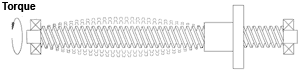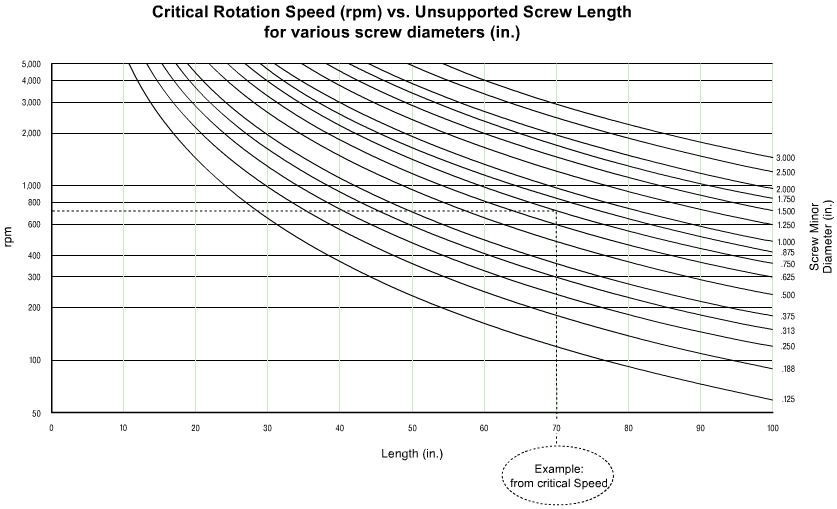## Speed Basics

Linear speed is dictated by the functional requirements of the application. Rotational speed is a function of the linear speed and the lead of the screw. Rotational speed (rpm) is equal to the linear speed (in./minute) divided by the lead of the screw (in./rev.). Leads are listed in the Screw/Nut Engineering Data for each screw series and size. For example, a 1 1/2 x .500 Hi-lead® screw is to move a load at 100 in./min. This will require a rotational input speed of 200 rpm (100 ipm/.500 ipr = 200 rpm).

### What is Critical Speed?

Critical speed is the engineering term for the first natural frequency of vibration of a rotating shaft.

Whether mounted horizontally or vertically, a rotating screw system must be operated below its critical speed to avoid:

• Vibration
• Noise
• Possible failure.

Critical speeds are shown in graphic form in Figure 27. Using the minor diameter of the screw from the Screw/Nut Engineering Data section for the selected screw and unsupported length of the screw, find the critical speed in rpm from the graph.

Using the formula for critical speed, the safe operating speeds can be calculated.

If your desired rpm is greater than the safe speed, increase the screw diameter, increase the screw lead (and decrease the rpm) or change the end fixity to provide more stiffness.

### Critical Speed Example

For example, a 1 x .333 Ball screw is selected to run at 200 in. per minute linear speed with a 70 in. span.

• The screw will have one end fixed and one end supported and a factor of safety of 2.0 will be used.
• Using the screw minor diameter for a 1 x .333 Ball screw of .75 in. from Table 26, the critical speed can be calculated from the formula or determined from the graph (Figure 28). Reading the graph for a minor diameter of .75 and a span length of 70 in., the critical speed is approximately 720 rpm.
• The safe operating speed is 558 rpm (720 x 1.55/2.0) where 1.55 is the correction factor for one end fixed and one end supported and 2 is the factor of safety.
• The rpm required for 200 in. per minute linear speed is 600 rpm (200 ipm/.333 in./rev.) where .333 is the screw lead.
• Since 600 rpm is greater than the safe operating speed of 558 rpm, a screw with a larger lead or diameter must be selected.
• Using the same desired conditions as above, a 1 x .500 Ball screw with a lead of .500 in./rev. will require only 400 rpm (200 ipm/.500 in./rev. = 400 rpm).
• Since 400 rpm is below the safe operating speed of 558 rpm, a 1 x .500 Ball screw will provide the desired linear speed at a safe operating rpm.

For data points beyond the range of the graphs, use the formula for Safe Operating Speed in the Useful Formulas section.FIGURE 27FIGURE 28